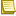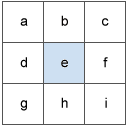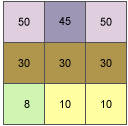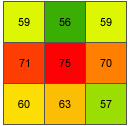Understanding the Neighborhood Slope Algorithm (also referred to as the average maximum technique)

When applying elevation data to produce slope angle, it is vital to understanding how the particular slope algorithm works. The most common algorithm is the neighborhood slope angle (used by ArcGIS and other GIS software; it is used to calculate slope for maps here).

The neighborhood slope algorithm or average maximum technique is explained on the ArcGIS website (outside link; opens in new tab).

Information from the ArcGIS website that can be accessed above that explains how the slope algorithm work is shown below:

The Slope algorithm

The rates of change (delta) of the surface in the horizontal (dz/dx) and vertical (dz/dy) directions from the center cell determine the slope. The basic algorithm used to calculate the slope is:

slope_radians = ATAN ( √ ([dz/dx]2 + [dz/dy]2) )

Slope is commonly measured in units of degrees, which uses the algorithm:

slope_degrees = ATAN ( √ ([dz/dx]2 + [dz/dy]2) ) * 57.29578Note:

The value 57.29578 shown here is a truncated version of the result from 180/pi.

The slope algorithm can also be interpreted as:

slope_degrees = ATAN (rise_run) * 57.29578
• where:
rise_run = √ ([dz/dx]2 + [dz/dy]2]

The values of the center cell and its eight neighbors determine the horizontal and vertical deltas. The neighbors are identified as letters from a to i, with e representing the cell for which the aspect is being calculated.Surface scanning window

The rate of change in the x direction for cell e is calculated with the following algorithm:

[dz/dx] = ((c + 2f + i) - (a + 2d + g) / (8 * x_cellsize)

The rate of change in the y direction for cell e is calculated with the following algorithm:

[dz/dy] = ((g + 2h + i) - (a + 2b + c)) / (8 * y_cellsize)

A slope calculation example

As an example, the slope value of the center cell of the moving window shown below will be calculated.Slope example input

The cell size is 5 units. The default slope measure of degrees will be used.

The rate of change in the x direction for the center cell e is:

[dz/dx] = ((c + 2f + i) - (a + 2d + g) / (8 * x_cellsize)
= ((50 + 60 + 10) - (50 + 60 + 8)) / (8 * 5)
= (120 - 118) / 40
= 0.05

The rate of change in the y direction for cell e is:

[dz/dy] = ((g + 2h + i) - (a + 2b + c)) / (8 * y_cellsize)
= ((8 + 20 + 10) - (50 + 90 + 50)) / (8 * 5)
= (38 - 190 ) / 40
= -3.8

Taking the rate of change in the x and y direction, the slope for the center cell e is calculated using:

rise_run = √ ([dz/dx]2 + [dz/dy]2)
= √ ((0.05)2 + (-3.8)2)
= √ (0.0025 + 14.44)
= 3.80032
slope_degrees = ATAN (rise_run) * 57.29578
= ATAN (3.80032) * 57.29578
= 1.31349 * 57.29578
= 75.25762

The integer slope value for cell e is 75 degrees.Slope example output

References

Burrough, P.A. and R.A. McDonell. 1998. Principles of Geographical Information Systems. Oxford University Press, New York.